# Mandelbrot Artists

Some artists working with the Mandelbrot set, and analysis of their artworks.

## 1 DinkydauSet

Gallery

### 1.1 Evolution Of Trees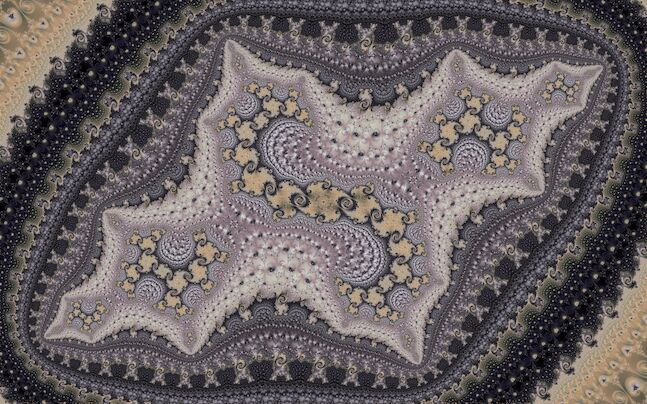Source

Coordinates

2013-09-25

#### 1.1.1 Analysis

Nearby periods:

• 1
• 93
• 145 (island in Seahorse Valley)
• 3913 (embedded Julia set in island’s Seahorse Valley)
• 4348 (starting to make a tree)
• 8841 (continuing to make a tree)
• 17628 (nearby but outside the tree)
• 26469 (one copy of the tree)
• 35455 (making tree)
• 61924 (one copy of the tree)
• 70910 (making tree)
• 106365 (one copy of the tree)
• 141965 (making tree)
• 212875 (one copy of the tree)
• 283930 (making tree)
• 425895 (one copy of the tree)
• 567135 (making an S out of the tree, final image center)
• 851065 (one copy of the tree, for final framing and alignment)

Final periods are far too high to be able to trace rays, even with perturbed algorithms.

$1 \frac{47}{93} 93 \frac{1}{2} 95 \frac{1}{2} 97 \frac{1}{2} 99 \frac{1}{2} 101 \frac{1}{2} 103 \\ \frac{1}{2} 105 \frac{1}{2} 107 \frac{1}{2} 109 \frac{1}{2} 111 \frac{1}{2} 113 \frac{1}{2} 115 \frac{1}{2} 117 \frac{1}{2} 119 \frac{1}{2} 121 \frac{1}{2} 123 \\ \frac{1}{2} 125 \frac{1}{2} 127 \frac{1}{2} 129 \frac{1}{2} 131 \frac{1}{2} 133 \frac{1}{2} 135 \frac{1}{2} 137 \frac{1}{2} 139 \frac{1}{2} 141 \frac{1}{2} 143 \\ \frac{1}{2} 145 \frac{13}{25} 3625 \frac{1}{2} 3770 \frac{1}{2} 3913 \frac{1}{2} 4203 \frac{1}{2} 4348 \frac{2}{3} 8841$

Address of p17628 does not contain p8841, ending:

$\cdots 4348 \frac{2}{3} 12321 \frac{1}{2} 12466 \frac{1}{2} 12611 \frac{1}{2} 12754 \frac{1}{2} 13044 \frac{1}{2} 13189 \frac{1}{2} 17537 \frac{1}{2} 17628$

This means it is in the cusp.

Conjecture: the symmetric version in the antenna ends:

$4348 \frac{2}{3} 8841 \frac{1}{2} 17628$

#### 1.1.2 Reconstruction

Trying similar structures with lower periods, starting from embedded Julia set near p3 island.

• 1
• 2
• 3
• 14/29 (Seahorse Valley)
• 86 (embedded Julia set)
• 95 (starting to make a tree)
• 193 (continuing to make a tree)
• 378 (nearby but outside the tree)
• 571 (one copy of the tree)
• 767 (making tree)
• 1338 (one copy of the tree)
• 1534 (making tree)
• 2301 (one copy of the tree)
• 3071 (making tree)
• 4605 (one copy of the tree)
• 6142 (making tree)
• 9213 (one copy of the tree)
• 12287 (making tree)
• 18429 (one copy of the tree)
• 24556 (making an S out of the tree, final image center)
• 36843 (one copy of the tree, for final framing and alignment)

The pattern of periods in the main sequence alternates between two recurrences: $p(n) = p(n-1) + p(n-3)$ $p(n) = p(n-1) + p(n-4) + 3$

The angled internal address (when choosing the antenna side of all symmetric choices) follows this pattern:

$1 \frac{1}{2} 2 \frac{1}{2} 3 \frac{14}{29} 86 \frac{1}{2} 92 \frac{1}{2} 95 \frac{1}{3} 193 \frac{1}{2} 288 \frac{1}{2} \\ 378 \frac{1}{2} 571 \frac{1}{2} 767 \frac{1}{2} 1145 \frac{1}{2} 1338 \frac{1}{2} 1534 \frac{1}{2} 2301 \frac{1}{2} 3071 \frac{1}{2} \\ p \frac{1}{2} q \frac{1}{2} r \frac{1}{2} s \frac{1}{2} \\ (s+q) \frac{1}{2} ((s+q)+q+3) \frac{1}{2} \cdots$

The S construction step is in the cusp of p18429: $\cdots 12287 \frac{1}{2} 21500 \frac{1}{2} 23801 \frac{1}{2} 24179 \frac{1}{2} 24553 \frac{1}{2} 24556$

Its pattern remains to be investigated (for example how it generalizes to a different count of tree making steps).

Considering four symmetric p36843 variants, it took 1h30m each to trace their rays outwards with perturbation. Then 10secs each to convert binary angle to address. The angles differ after p9213, the shortest angled address ends $\cdots 9213 \frac{1}{2} 12287 \frac{1}{2} 21500 \frac{1}{2} 23801 \frac{1}{2} 24179 \frac{1}{2} 24553 \frac{1}{2} 24556 \frac{1}{2} 36843$

#### 1.1.3 Variations

Varying the numerator of 14/29 in the reconstruction gives a family of 28 tree evolutions. Converting the address to a binary angle took 10mins each. Then tracing the p36843 rays inwards took up to 35mins each. Only 6 of the 28 succeeded, the others failed at varying depths, with reasons to be investigated. Rendering the 6 images took 50secs each: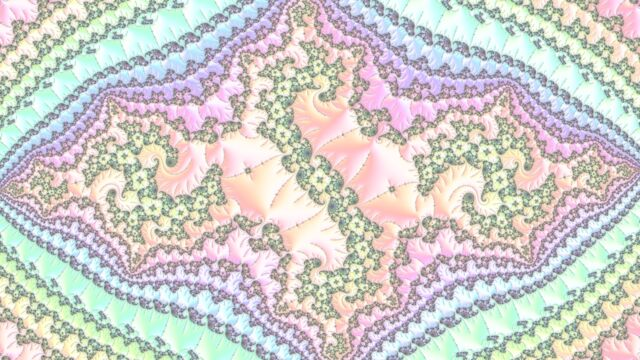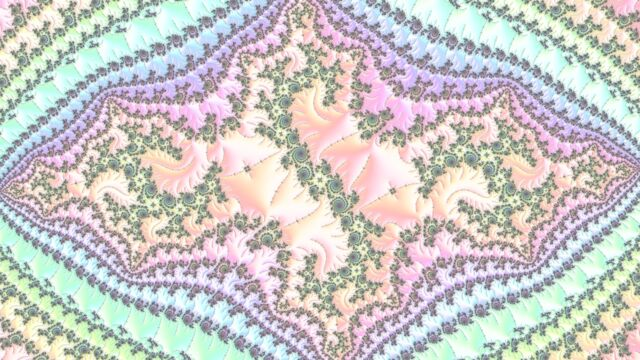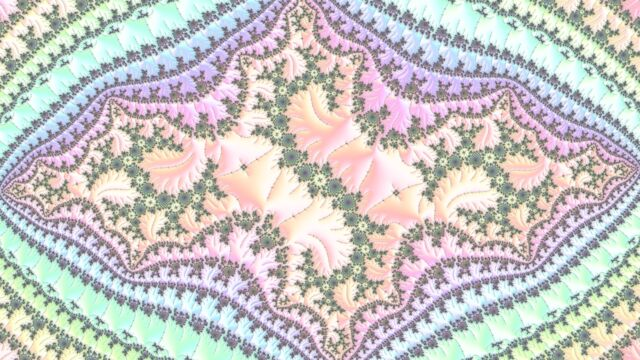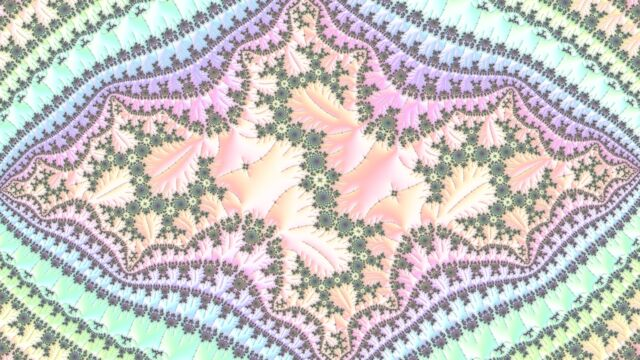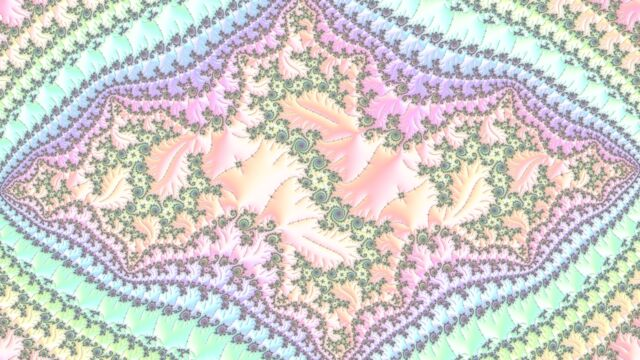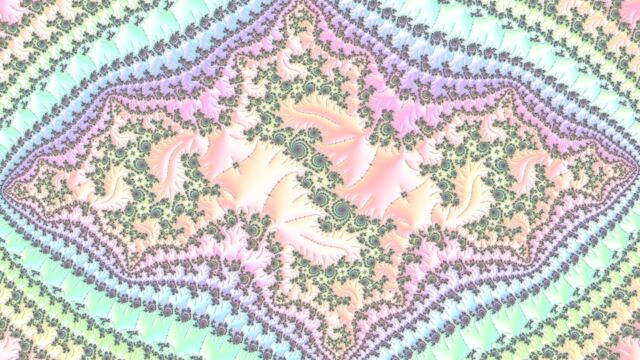### 1.2 Parallel Evolution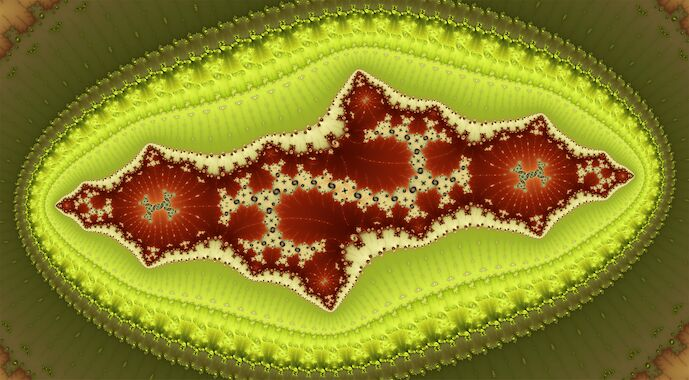Source

Coordinates

2014-05-07

#### 1.2.1 Recreation

Explored by hand in m-perturbator-gtk using “find nucleus” and “zoom to domain” features.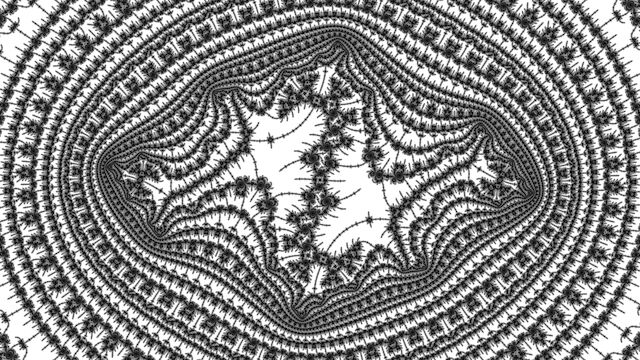Parameters: m-perturbator-gtk / fraktaler-3

Session with m-perturbator-gtk: video (1920x1080, 22MB).

### 1.3 3rd Order Evolution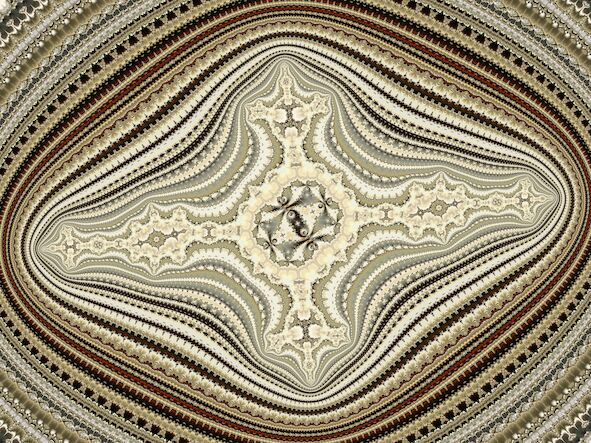Source

Coordinates

2014-12-25

#### 1.3.1 Analysis

• (image) 1.50e-000 : p1 to p2
• (image) 1.13e-000 : p2 to p3
• (image) 2.70e-002 : p3 to p6
• (image) 6.56e-003 : p6 to p120, 1/20
• (image) 2.21e-004 : p120 to p113, primary hair
• (image) 1.27e-006 : p113 to p122, tree (sideways in embedded Julia set)
• (image) 6.34e-009 : p122 to p243, evolution (nearby but outside tree)
• (image) 1.52e-010 : p243 to p252, stripe (long tip in embedded Julia set)
• (image) 1.55e-014 : p252 to p374, neighbouring tree
• (image) 9.34e-015 : p374 to p492, evolution (nearby but outside tree)
• (image) 3.36e-017 : p492 to p606, swirl (inside spiral in embedded Julia set)
• (image) 3.80e-023 : p606 to p980, neighbouring tree
• (image) 6.19e-024 : p980 to p1105, tree
• (image) 3.26e-034 : p1105 to p1963, neighbouring stripe
• (image) 5.29e-036 : p1963 to p2206, stripe
• (image) 4.20e-052 : p2206 to p3917, neighbouring swirl
• (image) 5.16e-054 : p3917 to p4637, swirl
• (image) 1.49e-078 : p4637 to p7948, neighbouring tree
• (image) 2.42e-080 : p7948 to p9056, tree
• (image) 9.27e-119 : p9056 to p15899, neighbouring stripe
• (image) 8.04e-120 : p15899 to p17862, stripe
• (image) 5.20e-174 : p17862 to p31555, neighbouring swirl
• (image) 2.42e-176 : p31555 to p36306, swirl
• (image) 3.06e-262 : p36306 to p63224, neighbouring tree
• (image) 9.97e-264 : p63224 to p72283, tree
• (image) 1.43e-393 : p72283 to p126451, neighbouring stripe
• (image) 3.70e-395 : p126451 to p142350, stripe
• (image) 1.82e-574 : p142350 to p250939, neighbouring swirl
• (image) 3.36e-576 : p250939 to p287359, swirl
• (image) 8.33e-862 : p287359 to p429709, final alignment

#### 1.3.2 Variation

Explored by hand in m-perturbator-gtk using “find nucleus” and “zoom to domain” features.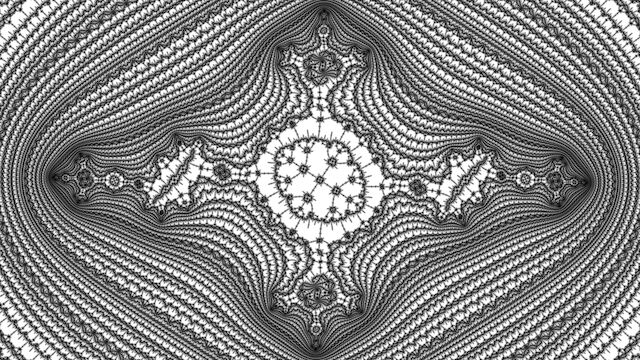Parameters: m-perturbator-gtk / fraktaler-3

Gallery

## 3 JWM

Gallery (mirror)

Software (mirror)

2021 blog post

Gallery

## 5 Olbaid-ST

Gallery

### 5.1 Deep Mandelbrot Set 001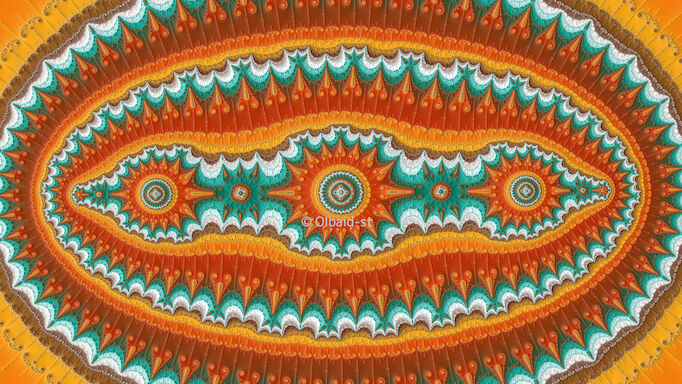Source

Coordinates

#### 5.1.1 Analysis

• p3 island, $(.(lo_3), .(hi_3)) = (.(011), .(100))$
• p38 in cusp, $(.(lo_{38}), .(hi_{38})) = (.(lo_3^{12}01), .(hi_3^{12}10))$
• p215 in 1/2, $(.(lo_{215}), .(hi_{215})) = (.(lo_{38}hi_{38}^{4}hi_{3}^{8}1), .(hi_{38}lo_{38}^{4}lo_{3}^{8}0))$ this step picks an island in the hairs
• 2-fold ejs: p253 in 1/2, p291 in cusp, $(.(lo_{291}), .(hi_{291})) = (.(lo_{215}hi_{38}lo_{38}), .(hi_{215}lo_{38}hi_{38}))$
• 2-fold ejs: p544 in 1/3, p620 in cusp, p658 in cusp, $(.(lo_{658}), .(hi_{658})) = (.(lo_{291}lo_{291}hi_{38}lo_{38}), .(lo_{291}hi_{291}lo_{38}hi_{38}))$
• 2-fold ejs: p911 in 2/3, p987 in cusp, p1025 in cusp, $(.(lo_{1025}), .(hi_{1025})) = (.(hi_{658}lo_{291}hi_{38}lo_{38}), .(hi_{658}hi_{291}lo_{38}hi_{38}))$
• 4-fold ejs: p2708 in 1/2, $(.(lo_{2708}), .(hi_{2708})) = (.(hi_{1025}lo_{1025}lo_{658}), .(hi_{1025}lo_{1025}hi_{658}))$ this step increases symmetry of central emblem
• 2-fold ejs: p3733 in 1/2, $(.(lo_{3733}), .(hi_{3733})) = (.(lo_{2708}hi_{1025}), .(hi_{2708}lo_{1025}))$ this step makes a row of three emblems
• 2-fold ejs: p6441 in 1/2, p7466 in 1/2, $(.(lo_{7466}), .(hi_{7466})) = (.(lo_{3733}lo_{2708}lo_{1025}), .(lo_{3733}hi_{2708}hi_{1025}))$ this step extends the row
• 2-fold ejs: p11199 in 1/2 frames final view (center p7466), $(.(lo_{11199}), .(hi_{11199})) = (.(lo_{7466}lo_{3733}), .(hi_{7466}hi_{3733}))$

#### 5.1.2 Reconstruction And Variations

Vary the depth of the (previously) p215 transition, by changing the exponent $$4$$ in $$(.(lo_{38}hi_{38}^{4}hi_{3}^{8}1), .(hi_{38}lo_{38}^{4}lo_{3}^{8}0))$$. Example ping-pong animation:

Vary the angle of the hair chosen in the third step (p215). Let $(.(o), .(i)) = (.(lo_{3}, hi_{3}))$ and $(.(O), .(I)) = (.(lo_{38}, hi_{38}))$ Then $$n \pmod{2^{d}}$$ expressed in binary with digits replaced by blocks $$O, I$$ ($$d = 5, n = 15, k = 8$$ for original p215): $(.(lo), .(hi)) = (.((n+0)_{O,I}^{d} i^{k} 1), .((n+1)_{O,I}^{d} o^{k} 0))$ Note a special case for cusp hair (ray order is swapped): $(.(O^d o^k 0), .(I^d i^k 1))$ Example ping-pong animation:

This second animation is incomplete: I wanted a full circuit of the p38 minibrot, instead of this ping-pong near the needle, but some of the islands gave bad results; I found out that my conjectures in the analysis above are false in general.

A more principled way to proceed using angled internal addresses was more successful: construct the p215 island angles as above, then convert to angled internal addresses, and graft a suffix to the address: $215 \frac{1}{2} 291 \frac{x}{3} 658 \frac{1}{2} 1025 \frac{1}{2} 2050 \frac{1}{2} 2708 \frac{1}{2} 3733 \frac{1}{2} 6441 \frac{1}{2} 7466 \frac{1}{2} 11199$ where x is 1 or 2. (Note: the original location has a slightly different suffix; this version is biased to the antenna side of symmetrically equivalent periods.) This gave 62 locations (no cusp hair) that I assembled into this video:

Unfortunately it is not as smooth as I had hoped for.

### 5.2 Deep Mandelbrot Set 003Source

Coordinates

#### 5.2.1 Periods

Using m-kfr-describe.sh from my mandelbrot-numerics library, the influencing periods are:

• a period 1 cardioid
• a period 2 circle
• a period 3 cardioid
• a period 6 circle
• a period 12 circle
• a period 171 cardioid
• a period 342 cardioid
• a period 690 cardioid
• a period 1386 cardioid
• a period 2082 cardioid
• a period 3474 cardioid
• a period 6954 cardioid
• a period 10434 cardioid
• a period 17394 cardioid
• a period 27828 cardioid
• a period 55656 cardioid

They are connected in the following way:

55656 = 27828 * 2
27828 = 17394 + 10434
17394 = 10434 + 6954 + 6
10434 = 6954 + 3474 + 6
6954 = 3474 * 2 + 6
3474 = 2082 + 1386 + 6
2082 = 1386 + 690 + 6
1386 = 690 * 2 + 6
690 = 342 * 2 + 6
342 = 171 * 2


#### 5.2.2 Conjectures

I suspect it may have been intended for each tree building step to be $p_n = p_{n-1} + p_{n-2} + 6$ with 1386 and 6954 anomalous.

An alternative could be for each tree building step to be $p_n = 2 p_{n-1} + 6$ which is as deep as possible maintaining 2-fold rotational symmetry.

The last two steps make a row of 3 shapes.

#### 5.2.3 External Angles

The external angles follow a pattern after p342.

p690  = (lo342  lo342  100 100, lo342  hi342  011 011)
p1386 = (hi690  hi690  011 011, hi690  lo690  100 100)
p2082 = (lo1386 lo690  100 100, lo1386 hi690  011 011)
p3474 = (lo2082 lo1386 011 011, lo2082 hi1386 100 100)


Watching the video and recreating the final two steps in a simpler example with low periods to trace rays, led to these patterns:

Penultimate step:

• Choice 1 (inside): (lo(n)lo(n-1), hi(n)hi(n-1))
• Choice 2 (outside): (lo(n)hi(n-1), hi(n)lo(n-1))

Final step:

• Choice 1 (inside): (lo(n)lo(n-1)lo(n-2), hi(n)hi(n-1)hi(n-2))
• Choice 2 (outside): (lo(n)hi(n-1)hi(n-2), hi(n)lo(n-1)lo(n-2))

The final two steps to make a row of three features makes the evolution of pairs of external angles have a couple of choices at each stage due to the symmetry: the difference is that one is inside and the other is outside the central minibrot considering paths along filaments coming from the period 1 continent.

The p to 2p+6 step has two choices too, whether to left or right (corresponding to internal angles 1/3 or 2/3 in the address):

• Choice 1 (1/3): (lo(n)lo(n)100100, lo(n)hi(n)011011)
• Choice 2 (2/3): (hi(n)lo(n)100100, hi(n)hi(n)011011)

The p q to q+p+6 step also has two choices:

• Choice 1 (1/3): (lo(n)lo(n-1)100100, lo(n)hi(n-1)011011)
• Choice 2 (2/3): (hi(n)lo(n-1)100100, hi(n)hi(n-1)011011)

Finding the address from the external angle using my mandelbrot-symbolics library:

1 2 3 6 12 14/15 171 2/3 336 339 342 1/3 687 690 2/3 1383 1386 2079 2082


The $$p_n = 2 p_{n-1} + 6$$ step seems to be p x/3 (2p+3) (2p+6) and the $$p_n = p_{n-1} + p_{n-2} + 6$$ step seems to be p ... q (q+p+3) (q+p+6).

#### 5.2.5 Reconstruction And Variations

Start with the above address until p342. Keep track of pairs of external angles. Do p to 2p+6 steps several times, followed by the final 2 steps. Trace the final ray. Align by finding a nearby q+p nucleus at distance atom domain size to the power of 9/8.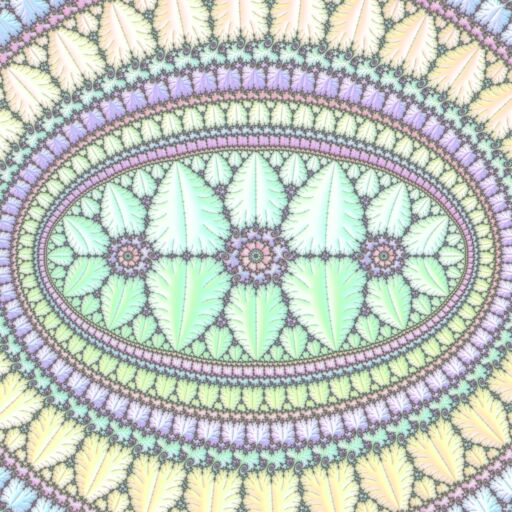Parameters

As above with p q to q+p+6 steps. Do one p to 2p+6 step first to initialize. Notice the different density of decorations around the central feature.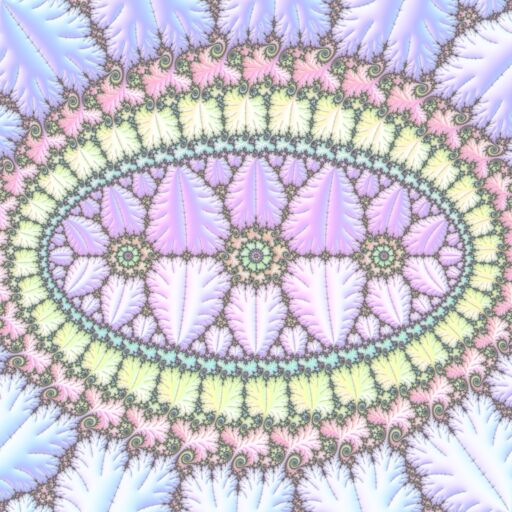Parameters

As above with branches of length k=5 instead of 2 (this is the number of times 011 and 100 are appended, the steps become p to 2p+3k and p q to q+p+3k).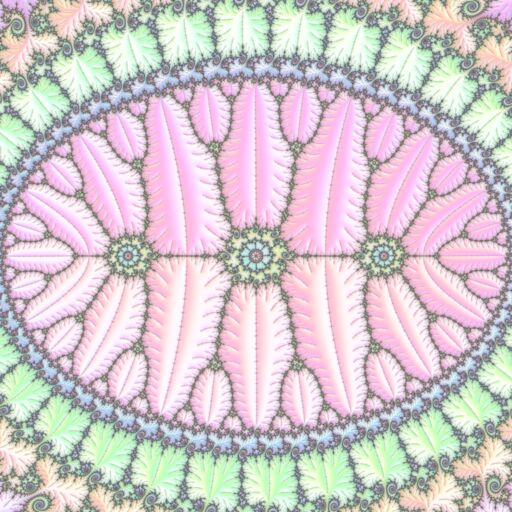Parameters

Another variation can be to choose different numerators for the internal angles at each stage.

Another variation can be to choose a different embedded Julia set near the period 3 influencing island.

Finally, start from a different influencing island (use its angles instead of the 011, 100 of the period 3 island) and embedded Julia set.

### 5.3 Deep Mandelbrot Set 052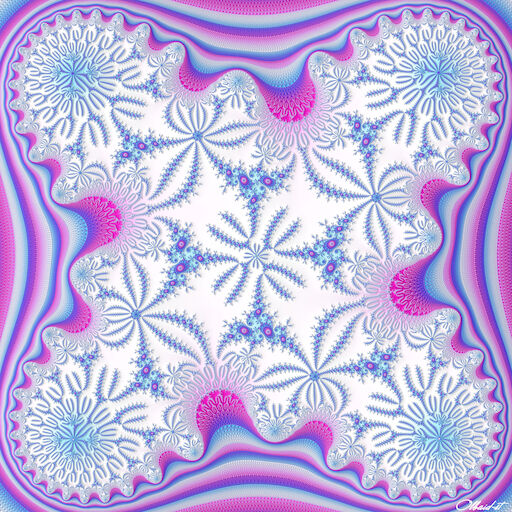Source

Coordinates

#### 5.3.1 Analysis

Parameters: m-perturbator-gtk

#### 5.3.2 Variation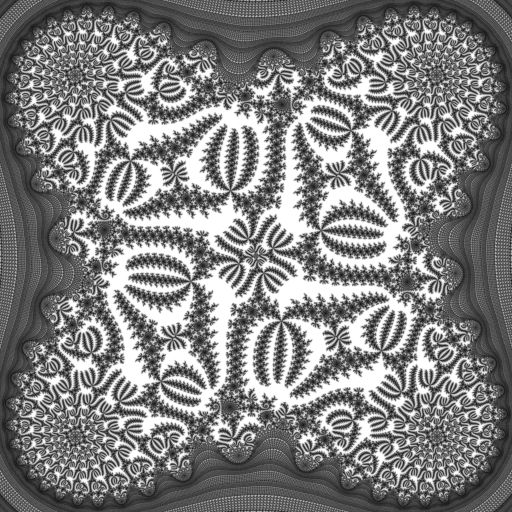Parameters: m-perturbator-gtk / fraktaler-3

Process: